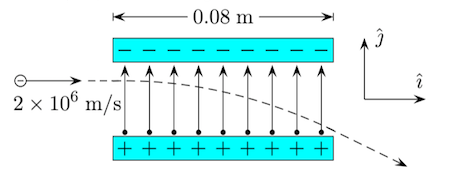# Problem: An electron traveling at 2 × 106 m/s enters a 0.08 m region with a uniform electric field of 246 N/C , as in the figure. A) Find the magnitude of the acceleration of the electron while in the electric field. The mass of an electron is 9.109 × 10−31 kg and the fundamental charge is 1.602 × 10−19 C . Answer in units of m/s2. B) Find the time it takes the electron to travel through the region of the electric field, assuming it doesn’t hit the side walls. Answer in units of s. C) What is the magnitude of the vertical displacement ∆y of the electron while it is in the electric field? Answer in units of m.

###### FREE Expert Solution
92% (410 ratings)
###### Problem Details

An electron traveling at 2 × 106 m/s enters a 0.08 m region with a uniform electric field of 246 N/C , as in the figure.

A) Find the magnitude of the acceleration of the electron while in the electric field. The mass of an electron is 9.109 × 10−31 kg and the fundamental charge is 1.602 × 10−19 C . Answer in units of m/s2.

B) Find the time it takes the electron to travel through the region of the electric field, assuming it doesn’t hit the side walls. Answer in units of s.

C) What is the magnitude of the vertical displacement ∆y of the electron while it is in the electric field? Answer in units of m.